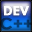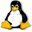Home Latest News Archive Tutorials C++ 2 3 4 Win32 Java OpenGL GLUT OpenGL ES 2 3 4 DirectX VB.NET HTML Photoshop 3dsmax Forums Projects Modelling Links About Us Contact Search Recent Tutorials C / C++ Win32 OpenGL OpenGL ES GLUT## String-Number Conversions

Tutorials > C++ > String-Number Conversions

### Introduction

You may find that you have a value stored in a string, but you would actually like it converted to an integer. It could work the other way around that you have a number and would like to convert it to a string. This tutorial will show you how to achieve this.

Contents of main.cpp :

```#include <iostream>
#include <stdlib.h>
```

String Streams are used in this tutorial and therefore the include is given below.

```#include <sstream>

using namespace std;

int main()
{

// C Conversions
//---------------
char *cstrval = "65";
char cstrval2;
int cval = 0;
```

To convert a character string to an integer in C, you can use the atoi (ascii to integer) function. This function takes the character string as its only parameter and returns the integer value. If the conversion cannot be made, 0 is returned. Other functions such as atof and atol also exist for different data types eg. float, long, etc.

In Unix and Linux, the sprintf function can be used instead which takes the storage string as the first parameter, the format of the string as the second parameter and other variables as the following parameters following the same pattern as the format. This is similar to the printf function.

```	cval = atoi(cstrval);
cout << cval << endl;
```

The opposite of this function is the itoa (integer to ascii) function. This function takes 3 parameters. The first is the number that you want to convert. The second parameter is the storage for the resulting character string and the third parameter indicates what base we are dealing with. We are dealing with base 10 and so we therefore use 10 as the third parameter.

```	itoa(cval, cstrval2, 10);
cout << cstrval2 << endl;
```

C++ string conversions take a bit more work. Data can be effectively converted by using string streams.

```	// C++ Conversions
//-----------------
int cppval = 0;

string s = "32";
string s2 = "";
string s3 = "";
```

First we create a stringstream object and pass it the string "32".

```	stringstream ssout(s);
```

You can now use the redirection operators as per normal. This has the same effect as the atoi function.

```
ssout >> cppval;

cout << cppval << endl;
```

Once again we are going to create a stringstream object. This object is created slightly differently as an extra parameter is given. This declares the mode that the stringstream object should follow.

We can pass a value of ios_base::in or ios_base::out. If no parameter is specified, ios_base::in is used. This specifies if you are passing information from a variable in to the stringstream object or if you are using the stringstream to pass data out of the stringstream object.

We are going to be converting an integer to a string and therefore we want to get the value stored in the stringstream object out.

```	stringstream ssin(s2, ios_base::out);
```

The next step is to pass the integer value into the stringstream object.

```	ssin << cppval;
```

Next we use the str method. This method returns the string that is currently stored within the stringstream object. This string should now contain our integer.

```	s2 = ssin.str();
cout << s2 << endl;
```

If you want to use both ios_base::in and ios_base::out properties, you can separate them by an | symbol. This allows you to insert a string into the stringstream and retrieve it again.

```	stringstream ssboth(s3, ios_base::in | ios_base::out);

ssboth << "Hello";
ssboth >> s2;

cout << ssboth.str() << endl;
cout << s2 << endl;

system("pause");

return 0;
}```

You should now be able to convert between numbers and strings easily. Remember that if you want, you can even use the atoi and itoa functions with C++ strings if you use the c_str method of the string object.

Please let me know of any comments you may have : Contact Me

 Source Files :< Tutorial 42 - Dynamic Matrices Tutorial 44 - Namespaces >

All Rights Reserved, © Zeus Communication, Multimedia & Development 2004-2005

Read the Disclaimer